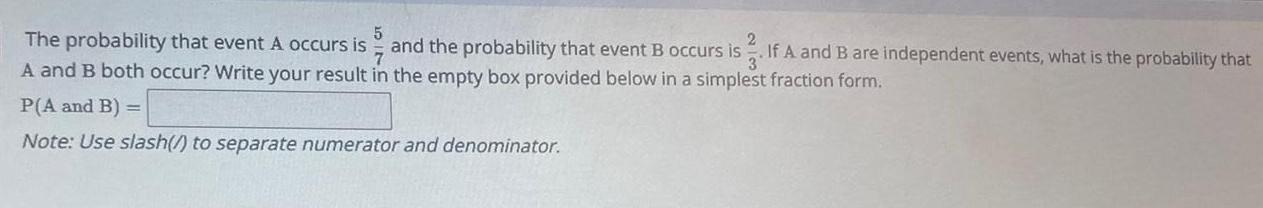Question:

# 5 The probability that event A occurs is 7 A and B both

Last updated: 9/7/20235 The probability that event A occurs is 7 A and B both occur Write your result in the empty box provided below in a simplest fraction form P A and B Note Use slash to separate numerator and denominator 2 and the probability that event B occurs is If A and B are independent events what is the probability that 3